# 第一周内容总结

1. 任务1：刷洛谷题
2. 任务2：继续学习C语言
3. 任务3：熟悉实验室环境

### 任务1 刷洛谷题：

1. 详情：12月3日前，洛谷题单“入门1”到“入门6”中所有小于P2000的题
2. 完成度：90%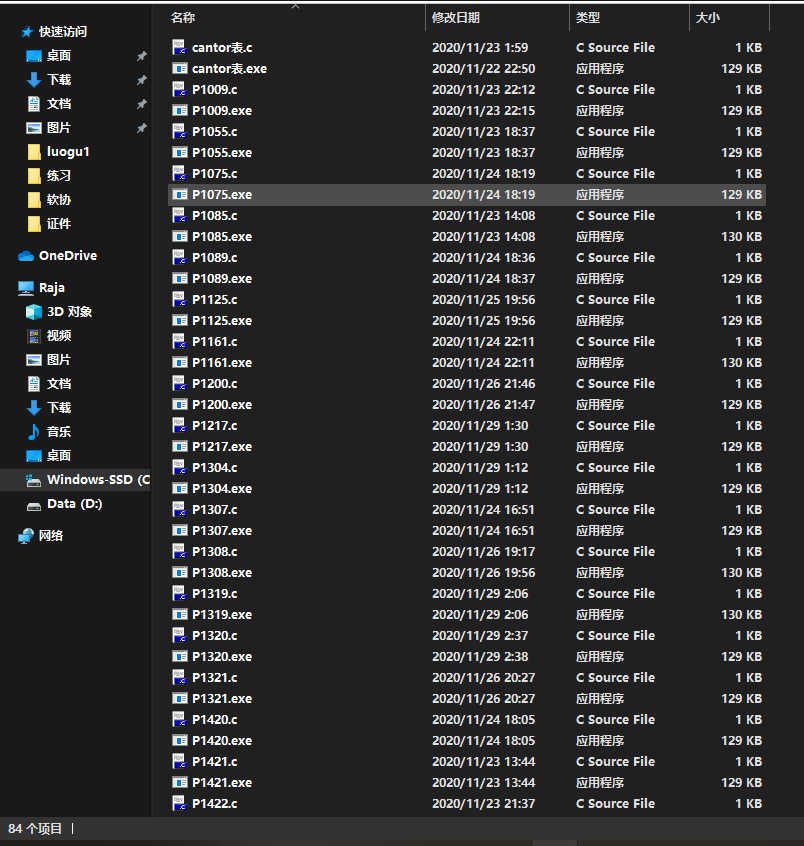3. 心得：题目很经典，有难有易，十分考验基础知识的把握能力和编程能力。
做这些题的过程中我发现了很多很细微却很重要的知识点。
比如数组在创建之后一定要初始化，否则可能会导致你的结果出现很多奇奇怪怪的值。
亦或者在使用数组时一定要注意数组的范围，不然很容易导致程序崩溃。
以及如何获取一段未知长度的数据：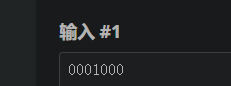while((scanf("%d",&n))!=EOF);//当scanf返回-1时停止循环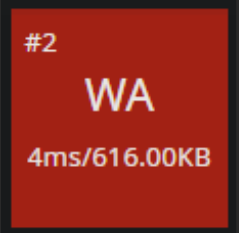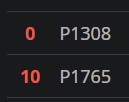### 任务2：继续学习C语言char* getss(char*p,int num){//这里我们定义了一个读取不读取回车的读取字符串函数
int i;
char c;
for(i=0;i<num;){
c=getchar();
if(c!='\n'){
p[i++]=c;
}else{
p[i]=0;
return p;
}
}
}


#include<stdio.h>
void x(int m,int n);
int main(void)
{
int m,n;
scanf("%d%d",&m,&n);
x(m,n);

return 0;
}
void x(int m,int n)
{
int p[m][n];
int count=0;
int a,b,c,d,e,f;
for(a=0,b=m-1,c=n-1;a<n;a++,b--,c--)
{
for(d=a;d<=c;d++)
{
count++;
p[a][d]=count;
//6printf("%d_1 ",p[a][d]);
if(count==m*n) goto next;
}
for(d=a+1;d<=b;d++)
{
count++;
p[d][c]=count;
//printf("%d_2 ",p[d][c]);
if(count==m*n) goto next;
}
for(e=c-1;e>=a;e--)
{
count++;
p[b][e]=count;
//printf("%d_3 ",p[b][e]);
if(count==m*n) goto next;
}
for(f=b-1;f>=(a+1);f--)
{
count++;
p[f][a]=count;
//printf("%d_4 ",p[f][a]);
if(count==m*n) goto next;
}
}
int g,h;
next:;
for(g=0;g<m;g++)
{
for(h=0;h<n;h++)
{
printf("%-4d",p[g][h]);
}
printf("\n");
}
}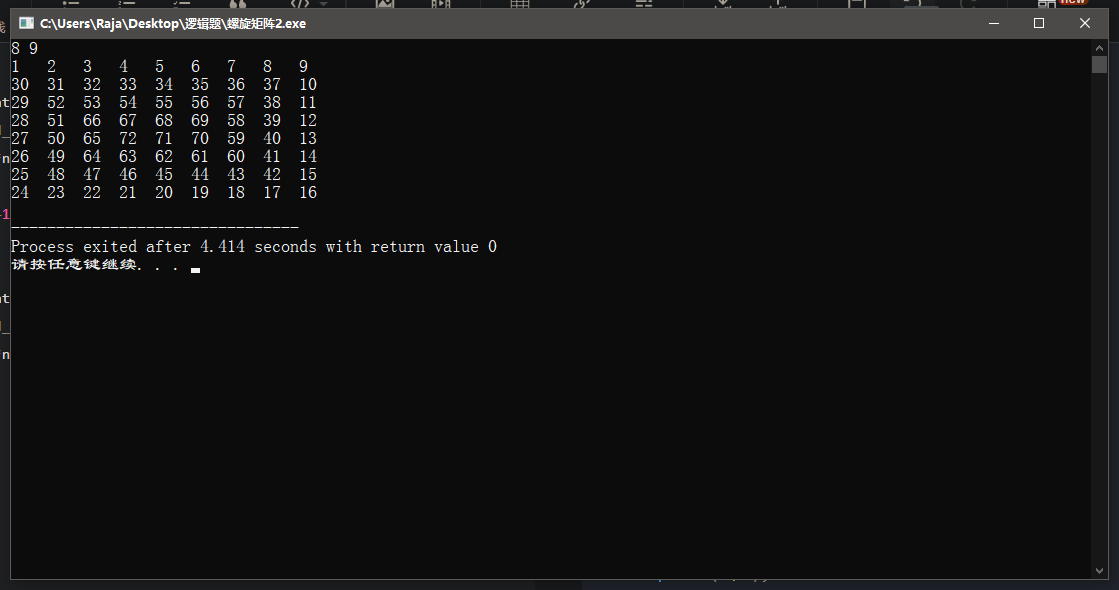## 目标

10-29348
04-1992912-2780
10-2586
01-20794
03-023570
07-081407
11-25656
11-182750
08-021992
02-162600
01-27440
12-043398
03-21597
04-20473
10-01919
03-071004
04-13605
01-081870